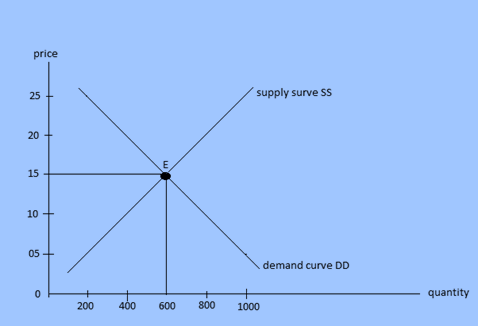# Equilibrium

In a competitive market the interaction of the forces of demand and supply determine the market price of any commodity.

Equilibrium is a condition where there is no tendency to change. Therefore, a competitive market attains equilibrium at that point where both buyers and sellers do not change the demanded quantity and supply quantity as well at the given price.

According to Alfred Marshall, ”As both blades if a scissor is important to cut a piece of cloth, so is demand and supply essential for the determination of price.”

It means that in a perfect competitive market, price of a commodity is determined at that point where quantity demanded is equal to quantity supplied. Interaction of demand and supply can be explained by the help of table and figure.

 Price of the commodity ( in Rs) Quantity demanded Quantity supplied balance 5 1000 200 D>S 10 800 400 D>S 15 600 600 D = S 20 400 800 D

This table shows the direct relationship between price and quantity supplies and opposite relation between price and quantity demanded. In the table, equilibrium price is Rs. 15 and equilibrium quantity is 600 kg. at Rs 5 and Rs 10, there is excess demand over supply because of low price of the commodity. As a result, there is pressure on price to rise so that the price increases to Rs 15 where demand is equal to supply. On the other hand at Rs 20 and Rs 25 there is excess supply over demand because of higher price of the commodity. As a result, there is pressure on price to fall. Therefore, price decreases and comes to Rs 15 where quantity demanded is equal to quantity supplied. The following diagram shows the interaction between demand and supply.In the given figure, x-axis represents quantity and y-axis represents price of the commodity.DD is demand curve and SS is supply curve. The marker is in equitation at point E where quantity demanded is equal to quantity supplies. Thus equilibrium price and output are determined at Rs 15 and 600 kg respectively. There will be excess supply over the price Rs 15 and pressure to fall unless the quantity demanded becomes equal to quantity supplied. Likewise, there will be excess demand over supply at price less than Rs 15 so there is pressure on price to rise unless and until the quantity demanded becomes equal to quantity supplied. Thus, in a perfect competitive market price is determined through the forces of market demand and supply of the commodity.